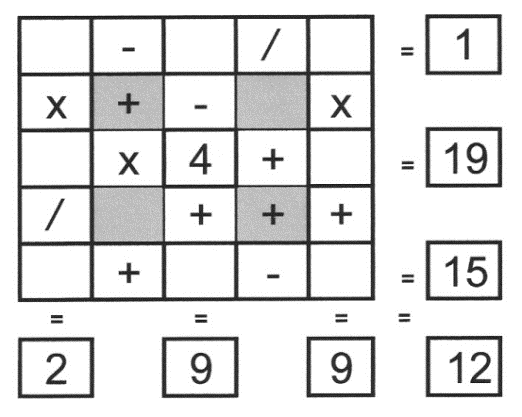Select Page

Each month, a new set of puzzles will be posted.  Come back next month for the solutions and a new set of puzzles, or subscribe to have them sent directly to you.

## MIND-Xpander maths problem

What ‘interesting’ value do you get when you sum together the square of the first seven prime numbers?

## Alphametic puzzles

Alphametic puzzles (sometimes known as Cryptarithms or Verbal Arithmetic), are puzzles where words or phrases are put together in a correct arithmetic expression such that numbers can be substituted for the letters to make the expression true. Find the numeric equivalent for each of the following alphametic expressions. Each letter is unique with its whole-number being 0 to 9. Note: there may be more than one solution.

CHECK + THE = TIRES, (Given: E = 4)

NO + NOT + THAT = AGAIN

## EQUATE+1 puzzle

Each row, column & diagonal is an equation and you use the numbers 1 to 9 to complete the equations. Each number can be used only once.  One number has been provided to get you started. Find the remaining eight numbers that satisfy all the resulting equations. Note – multiplication (x) & division (/) are performed before addition (+) and subtraction (-).## Feedback

There are more than one way of doing these puzzles and may well be more than one answer.  Please let me and others know what alternatives you find by commenting below.  We also welcome general comments on the subject and any feedback you'd like to give.

If you have a question that needs a response from me or you would like to contact me privately, please use the contact form.

## Get more puzzles!

If you've enjoyed doing the puzzles, consider ordering the book; 150+ of the best puzzles in a handy pocket sized format. Click here for full details.

## MIND-Xpander Maths Problem

If N is a whole number and the following limits apply: 2N > 30 and 5N < 100, what values for N are possible?

Lower limit: Where 2N > 30 or N > 15, then N must be ‘greater than’ 15. Upper limit: Where 5N < 100 or N < 20, then N must be ‘less than’ 20.

Therefore, the possible values for N between the lower and upper limits would be 16, 17, 18 and 19.

## Petite CIRCLE-Sums Puzzles

The number within the 4 sectors of the outer circle is equal to the sum of the three numbers in its sector. The numbers in the individual circles can only be 1 to 9 and each number can be used only once. One number has been provided to get you started. Find the remaining 4 numbers. Note: There may be more than one solution.## EQUATE+2 Puzzle

Each row, column & diagonal is an equation and you use the numbers 1 to 9 to complete the equations. Each number can be used only once. ‘Two’ numbers have been provided to get you started. Find the remaining seven numbers that satisfies all the resulting equations. Note – multiplication (x) & division (/) are performed before addition (+) and subtraction (-).